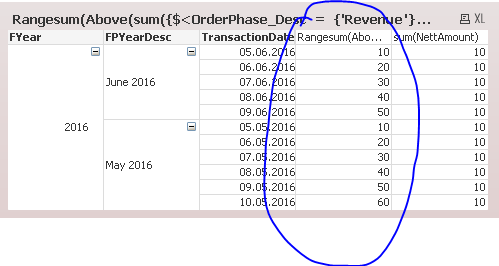# QlikView Layout & Visualizations

Discussion Board for collaboration on QlikView Layout & Visualizations.

HighlightedLuminary

## Aggr & Rangesum

Hi,

If the following formula:

Rangesum(Above(sum({\$<OrderPhase_Desc = {'Revenue'}>}NettAmount\$(vCurrency)), 0,rowno())))

The issue is that I use it in a Pivot table having three dimensions: FYear, FPYearDesc and Transaction Date. What I want is that the Rangesum calculates the sum only within a FPYearDesc (means a period). Or in other words an aggregation within one month like this:

Value of Day 1 = Day 1,

Value of Day 1+2 = Value Day 2

Values of Day 1+2+3 = Value Day 3

What it does now is it does a calculation over all values no matter which FPYearDesc. How do I need to adjust it? I tried it with Aggr, but then I don´t get the value in each line...only in the first line then.

Many thanks for your help!

1 Solution

Accepted SolutionsMVP

## Re: Aggr & Rangesum

Try this:

=If(Dimensionality() = 3, Rangesum(Above(sum({\$<OrderPhase_Desc = {'Revenue'}>}NettAmount), 0, RowNo())), Sum(NettAmount))

10 Replies
Honored Contributor III

## Re: Aggr & Rangesum

Try using Dimensionality() or total<Dim...> function or can you share small sample to try with.MVP

## Re: Aggr & Rangesum

Like digvijay‌ would you be able to share a sample with the expected output to provide you with better help?MVP

## Re: Aggr & Rangesum

How does your pivot table chart layout look like? Do you have any dimension pivoted? And which dimension is last and second to last w.r.t sort order?

In a pivot table,you might need to use another chart inter record function (e.g. Before() instead of Above() ) for pivoted dimensions.

And the chart inter record functions will consider column / row segments, so Transaction Date need to be the last in sort order to reset the Rangesum accordingly at segment bounderies.Luminary

## Re: Aggr & Rangesum

It is FYear / FPYearDesc / TransactionDate.Luminary

## Re: Aggr & Rangesum

I added a sample qvw now. Please see above.MVP

## Re: Aggr & Rangesum

I am still not sure what your desired output is, but is this what you want?Expression:

Rangesum(Above(sum({\$<OrderPhase_Desc = {'Revenue'}>}NettAmount), 0, RowNo()))MVP

## Re: Aggr & Rangesum

Hi Carolin, probably I misunderstood something, I tried your expression (adapted) and it seems to work.

Only issue I found is when some parts of the pivot table are colapsed and others expanded, I added a condition trying to check this:

If(IsNull(rowno()), Sum(NettAmount), Rangesum(Above(sum(NettAmount), 0,rowno())))

In this sample seems to work but in a more complex table it can failLuminary

## Re: Aggr & Rangesum

Very close. The formula is already correct. Is there a possibility to show 50 wen e.g. June 2016 is collapsed? And 110 when 2016 is collapsed?MVP

## Re: Aggr & Rangesum

Try this:

=If(Dimensionality() = 3, Rangesum(Above(sum({\$<OrderPhase_Desc = {'Revenue'}>}NettAmount), 0, RowNo())), Sum(NettAmount))